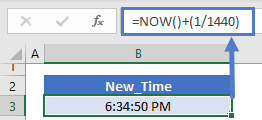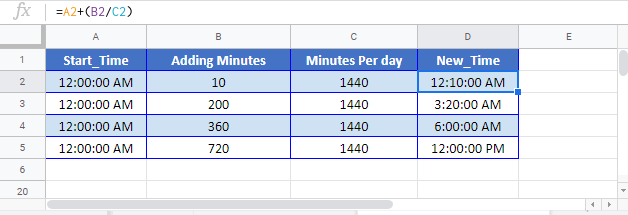This tutorial will demonstrate how to add minutes to a time in Excel and Google Sheets.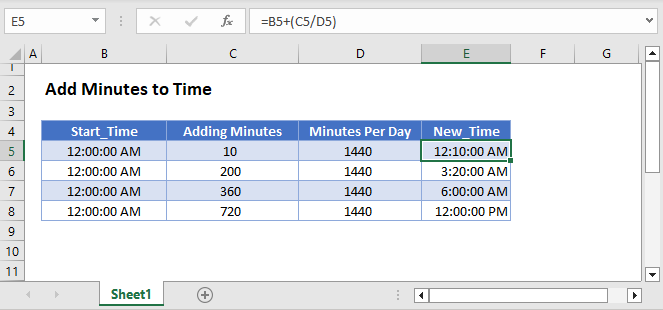In Excel, time is stored as a fractional value where each 1/24th represents one hour of the day. So each 1/1440 represents one minute of the day (24 hours * 60 minutes/hour = 1440 minutes).

``=B3/1440``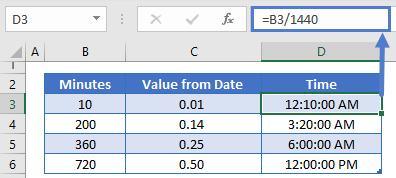``=B3+(1/1440)``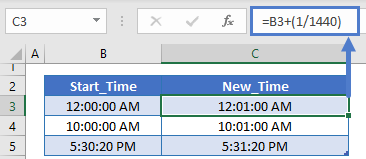or 59/1440 to add 59 minutes:

``=B3+(59/1440)``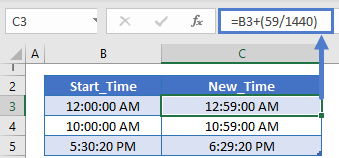To add a minute to the current time, add 1/1440 to the NOW Function:

``=NOW()+(1/1440)``